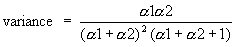The Rocscience International Conference 2021 Proceedings are now available. Read Now

# Beta Distribution

The Beta distribution is a very versatile function which can be used to model several different shapes of probability density curves, as shown in the figure below.

Beta (a1, a2) density functions. From Law (1991).The form of the Beta distribution is determined by the shape parameters a1 and a2. Both a1 and a2 are always > 0. The relationship between the Beta distribution shape parameters and the RocSupport input data is as follows:Eqn. 1.Eqn. 2.

The standard deviation is the positive square root of the variance.

Note that Equations 1 and 2 apply to a beta random variable in the range [0, 1]. To obtain a beta random variable on [a, b] of the same shape, use the transformation a + (b–a) * X formula to rescale and relocate.